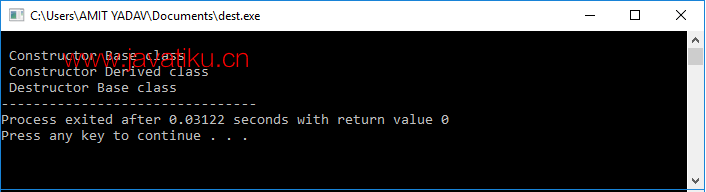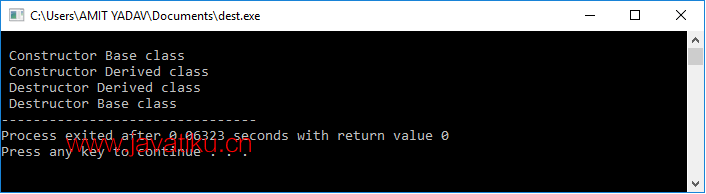# C++教程-C++中的虚析构函数## C++中的虚析构函数

### 编写一个在C++中不使用虚析构函数展示析构函数的未定义行为的程序。

``````#include<iostream>
using namespace std;
class Base
{
public:
Base() // 构造函数
{
cout << "\n Base类的构造函数";
}
~Base() // 析构函数
{
cout << "\n Base类的析构函数";
}
};

class Derived: public Base
{
public:
Derived() // 构造函数
{
cout << "\n Derived类的构造函数" ;
}
~Derived() // 析构函数
{
cout << "\n Derived类的析构函数" ; /* 不调用析构函数来释放其空间。 */
}
};
int main()
{
Base *bptr = new Derived; // 创建一个基类指针对象
delete bptr; /* 在这里调用指针对象来删除析构函数占用的空间。*/
}    ``````### 编写一个在C++中使用虚析构函数展示析构函数行为的程序。

``````#include<iostream>
using namespace std;
class Base
{
public:
Base() // 构造函数
{
cout << "\n Base类的构造函数";  // 首先输出
}
virtual ~Base() // 定义虚析构函数以调用派生类的析构函数
{
cout << "\n Base类的析构函数";
}
};
// 继承概念
class Derived: public Base
{
public:
Derived() // 构造函数
{
cout << "\n Derived类的构造函数" ; /* 在打印构造函数Base之后，现在会打印它。 */
}
~Derived() // 析构函数
{
cout << "\n Derived类的析构函数"; /* 虚基类的析构函数在调用基类的析构函数之前调用它。 */
}
};
int main()
{
Base *bptr = new Derived; // 一个指针对象引用基类
delete bptr; // 删除指针对象
}  ``````